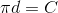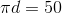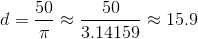# PSAT Math : How to find the length of the diameter

## Example Questions

### Example Question #1 : How To Find The Length Of The Diameter

If the area of a circle is four times larger than the circumference of that same circle, what is the diameter of the circle?

2

4

8

32

16

16

Explanation:

Set the area of the circle equal to four times the circumference πr2 = 4(2πr).

Cross out both π symbols and one r on each side leaves you with r = 4(2) so r = 8 and therefore = 16.

### Example Question #1 : Diameter

The perimeter of a circle is 36 π.  What is the diameter of the circle?

72

6

3

18

36

36

Explanation:

The perimeter of a circle = 2 πr = πd

Therefore d = 36

### Example Question #1 : How To Find The Length Of The Diameter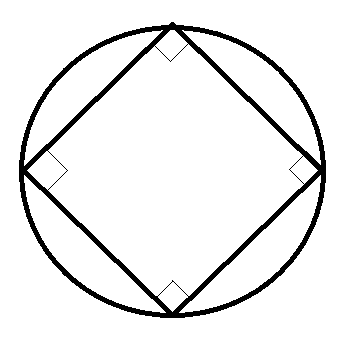If the area of the circle touching the square in the picture above is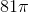, what is the closest value to the area of the square?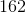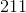Explanation:

Obtain the radius of the circle from the area.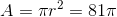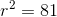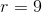Split the square up into 4 triangles by connecting opposite corners. These triangles will have a right angle at the center of the square, formed by two radii of the circle, and two 45-degree angles at the square's corners. Because you have a 45-45-90 triangle, you can calculate the sides of the triangles to be,, and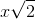. The radii of the circle (from the center to the corners of the square) will be 9. The hypotenuse (side of the square) must be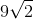.

The area of the square is then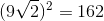.

### Example Question #1 : How To Find The Length Of The Diameter

Two legs of a right triangle measure 3 and 4, respectively. What is the area of the circle that circumscribes the triangle?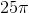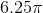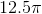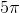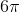Explanation:

For the circle to contain all 3 vertices, the hypotenuse must be the diameter of the circle. The hypotenuse, and therefore the diameter, is 5, since this must be a 3-4-5 right triangle.

The equation for the area of a circle is A = πr2.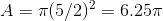### Example Question #2 : Diameter And Chords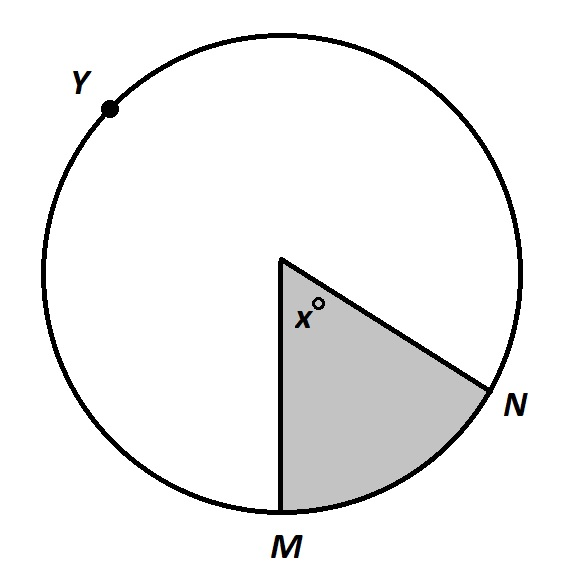Note: Figure NOT drawn to scale.

In the above circle, the length of arc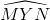is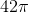, and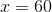. What is the diameter of the circle?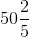Explanation:

Call the diameter. Since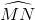is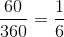of the circle, andis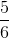of a circle with circumference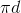.isin length, so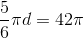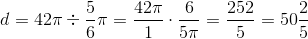### Example Question #3 : Diameter And ChordsNote: Figure NOT drawn to scale.

In the above circle, the length of arcis 10, and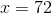. Give the diameter of the circle. (Nearest tenth).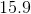Insufficient information exists to answer the question.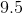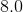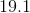Explanation:

Call the diameter. Sinceis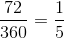of a circle with circumference. Since it is of length 10, the circumference of the circle is 5 times this, or 50. Therefore, set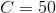in the circumference formula: